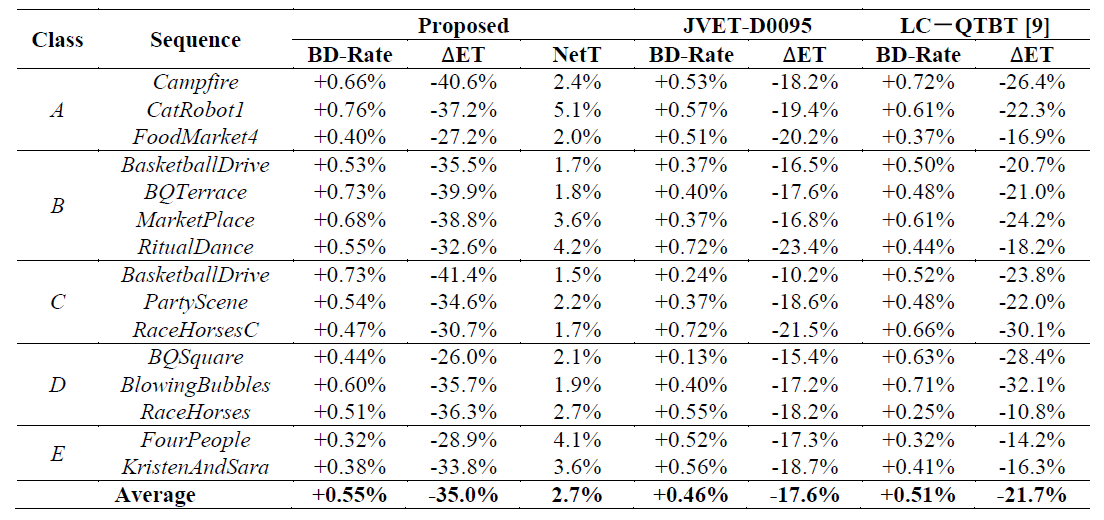# Reading: Wang ICIP’18 — Fast QTBT Partitioning Decision for Interframe Coding (Fast VVC Prediction)

## 35% Encoding Time Reduction With Only 0.55% Increase in Bitrate

this story, Fast QTBT Partitioning Decision for Interframe Coding with Convolution Neural Network (Wang ICIP’18), by Peking University, City University of Hong Kong, and University of Southern California, is presented. I read this because I work on video coding research. In this paper, a convolution neural network (CNN) oriented fast QTBT partitioning decision algorithm for inter coding is proposed. This is a paper in 2018 ICIP. (Sik-Ho Tsang @ Medium)

# Outline

1. QTBT Partition Depth Range as a Multi-Class Classification Problem
2. CNN Network Architecture & Some Training Details
3. VVC Implementation
4. Experimental Results

# 1. QTBT Partition Depth Range as a Multi-Class Classification Problem

## 1.1. Distribution of the maximal QTBT depth (MaxDepth) for 128×128

• The distribution of the maximal QTBT depth (MaxDepth) for 128×128 is as shown above.
• MaxDepth is found by finding the maximum depth for each sub-CU within a particular CU size, and in this case, 128×128 CU.
• It is found that the distribution for 128×128 is very imbalance which is not good for training.

## 1.2. Distribution of the maximal QTBT depth (MaxDepth) for 32×32

• The distribution of the maximal QTBT depth (MaxDepth) for 32×32 is as shown above.
• The class imbalance problem is much less compared to the 128×128 one.
• However, if 32×32 CU is used as basis for classification, there is no speed up at 64×64 which makes the performance inferior, just like Jin VCIP’17.

## 1.3. Distribution of the maximal QTBT depth (MaxDepth) for 64×64

• The distribution of the maximal QTBT depth (MaxDepth) for 64×64 is as shown above.
• The class imbalance problem is much less compared to the 128×128 one.
• And also, we can have the speed up for 64×64 CU.
• Finally, 64×64 CU is selected for CNN classification, which serves as the foundation of the proposed scheme.
• To avoid overfitting for the categories with scarce percentage, such as “1” and “3”, these categories are merged into the adjacent categories to generate more reliable data, as shown in the table above.
• With smaller class label number, texture becomes smooth/flat.
• With larger class label number, texture becomes complex.

# 2. CNN Network Architecture & Some Training Details

• The residual block is subtracted by the mean intensity values before input.
• After pre-processing, 4×4 kernels at the first convolutional layer is used to extract the low level features.
• For the second and third layers, feature maps are further convoluted twice with 2×2 kernels.
• The final feature maps are concatenated together and flatten into a vector. And the vector goes through the fully connected layers for classification.
• Standard multi-class label loss function is used:
• Training samples are collected with five sequences (BasketballPass, BQMall, Johnny, Cactus, and ParkRunning3) of different resolutions and characteristics. They were encoded with the JEM7.0 reference software.
• Moreover, samples for which there is little RD cost difference between the optimal result and non-splitting case are eliminated, since such samples may make the nets get trapped in ill-conditions during the network training.

# 3. VVC Implementation

1. The current CTU is divided into four 64×64 patches directly, and the MaxDepth 𝑑𝑖 (𝑖 = 0,1,2,3) is predicted for each patch with the CNN.
2. Same processing in Step 1 is applied to the colocated CTU in the reference frame. Thus, the actual and predicted MaxDepth of each co-located patch which are denoted as 𝐷𝑖′ and 𝑑𝑖′, can be obtained.
3. The predicted MaxDepth based on 𝐷𝑖′ and 𝑑𝑖′ is as:
• 𝑑𝑖 keeps unchanged when the co-located path is precisely predicted.
• If 𝑑𝑖′ is predicted to be larger than its actual value, 𝑑𝑖 of the current patch is also unchanged to ensure enough partitioning depth.
• Otherwise, when 𝑑𝑖′ is predicted to be smaller than its actual value, the prediction difference will be added to 𝑑𝑖′.

4. Within the 128×128 CU, if all (four) 𝑑𝑖 are zero, 128×128 CU conducts further partitions with depth less than 2, and the optimal shape will be selected.

• If only one 𝑑𝑖 ≠ 0, the 128×128 CU will conduct all iterations while the patches with 𝑑𝑖 = 0 will be early terminated.
• Otherwise, the CTU will be directly partitioned by QT and each 64×64 CU iterates according to its corresponding depth range.

# 4. Experimental ResultsBD-Rate (%) and Encoding Time Difference (ΔET) (%) Against the Conventional JEM-7.0 Using Random Access (RA) Configuration
• It is observed that an averaged 35% time saving can be achieved with 0.55% negligible BD-rate increase, and outperforms the two methods as shown in the table above.

During the days of coronavirus, A challenge of writing 30 stories again for this month has been accomplished. A new target of 35 stories is set by now. This is the 32nd story in this month.. Thanks for visiting my story..

## Reference

[2018 ICIP] [Wang ICIP’18]
Fast QTBT Partitioning Decision for Interframe Coding with Convolution Neural Network